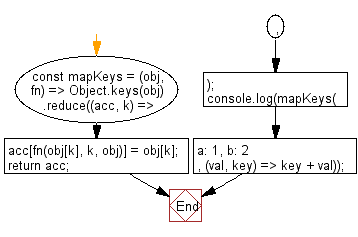# JavaScript: Create an object with keys generated by running the provided function for each key and the same values as the provided object

## JavaScript fundamental (ES6 Syntax): Exercise-81 with Solution

Write a JavaScript program to create an object with keys generated by running the provided function for each key and the same values as the provided object.

• Use Object.keys() to iterate over the object's keys.
• Use Array.prototype.reduce() to create a new object with the same values and mapped keys using fn.

Sample Solution:

JavaScript Code:

``````//#Source https://bit.ly/2neWfJ2
const mapKeys = (obj, fn) =>
Object.keys(obj).reduce((acc, k) => {
acc[fn(obj[k], k, obj)] = obj[k];
return acc;
}, {});
console.log(mapKeys({ a: 1, b: 2 }, (val, key) => key + val));
```
```

Sample Output:

```{"a1":1,"b2":2}
```

Flowchart:Live Demo:

See the Pen javascript-basic-exercise-81-1 by w3resource (@w3resource) on CodePen.

Improve this sample solution and post your code through Disqus

What is the difficulty level of this exercise?

Test your Programming skills with w3resource's quiz.

﻿

## JavaScript: Tips of the Day

Chunks an array into n smaller arrays

Example:

```const tips_chunkIntoN = (arr, n) => {
const size = Math.ceil(arr.length / n);
return Array.from({ length: n }, (v, i) =>
arr.slice(i * size, i * size + size)
);
}
console.log(tips_chunkIntoN([1, 2, 3, 4, 5, 6, 7,8], 4));
```

Output:

```[[1,2],[3,4],[5,6],[7,8]]
```